# Lamé curve

Jump to: navigation, search

A plane algebraic curve whose equation in rectangular Cartesian coordinates has the formwhere,andare coprime numbers,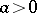and. The order of Lamé's curve is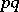if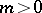and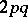if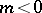. If, Lamé's curve is a straight line, ifit is an ellipse, and if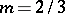and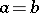it is an astroid. The Lamé curves are named after G. Lamé, who considered them in 1818.

How to Cite This Entry:
Lamé curve. Encyclopedia of Mathematics. URL: http://encyclopediaofmath.org/index.php?title=Lam%C3%A9_curve&oldid=22701
This article was adapted from an original article by D.D. Sokolov (originator), which appeared in Encyclopedia of Mathematics - ISBN 1402006098. See original article